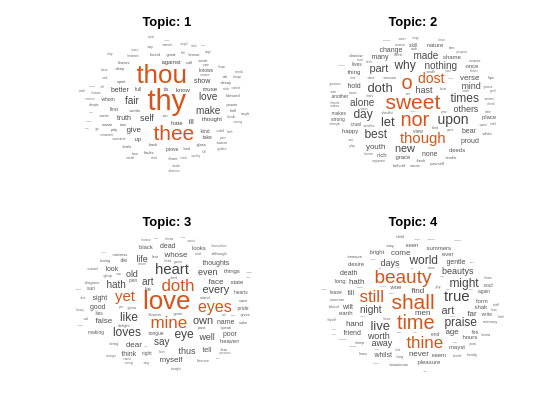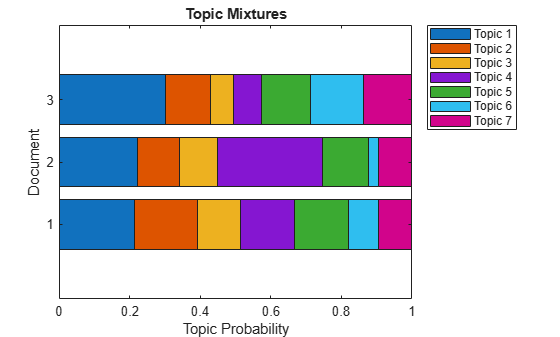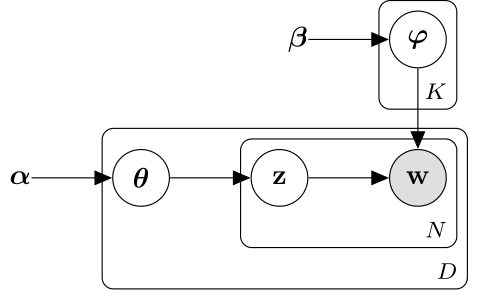Documentation

# fitlda

Fit latent Dirichlet allocation (LDA) model

## Syntax

``mdl = fitlda(bag,numTopics)``
``mdl = fitlda(counts,numTopics)``
``mdl = fitlda(___,Name,Value)``

## Description

A latent Dirichlet allocation (LDA) model is a topic model which discovers underlying topics in a collection of documents and infers word probabilities in topics. If the model was fit using a bag-of-n-grams model, then the software treats the n-grams as individual words.

example

````mdl = fitlda(bag,numTopics)` fits an LDA model with `numTopics` topics to the bag-of-words or bag-of-n-grams model `bag`.```

example

````mdl = fitlda(counts,numTopics)` fits an LDA model to the documents represented by a matrix of frequency counts.```
````mdl = fitlda(___,Name,Value)` specifies additional options using one or more name-value pair arguments.```

## Examples

collapse all

To reproduce the results in this example, set `rng` to `'default'`.

`rng('default')`

Load the example data. The file `sonnetsPreprocessed.txt` contains preprocessed versions of Shakespeare's sonnets. The file contains one sonnet per line, with words separated by a space. Extract the text from `sonnetsPreprocessed.txt`, split the text into documents at newline characters, and then tokenize the documents.

```filename = "sonnetsPreprocessed.txt"; str = extractFileText(filename); textData = split(str,newline); documents = tokenizedDocument(textData);```

Create a bag-of-words model using `bagOfWords`.

`bag = bagOfWords(documents)`
```bag = bagOfWords with properties: Counts: [154x3092 double] Vocabulary: [1x3092 string] NumWords: 3092 NumDocuments: 154 ```

Fit an LDA model with four topics.

```numTopics = 4; mdl = fitlda(bag,numTopics)```
```Initial topic assignments sampled in 0.2545 seconds. ===================================================================================== | Iteration | Time per | Relative | Training | Topic | Topic | | | iteration | change in | perplexity | concentration | concentration | | | (seconds) | log(L) | | | iterations | ===================================================================================== | 0 | 0.01 | | 1.215e+03 | 1.000 | 0 | | 1 | 0.02 | 1.0482e-02 | 1.128e+03 | 1.000 | 0 | | 2 | 0.02 | 1.7190e-03 | 1.115e+03 | 1.000 | 0 | | 3 | 0.02 | 4.3796e-04 | 1.118e+03 | 1.000 | 0 | | 4 | 0.01 | 9.4193e-04 | 1.111e+03 | 1.000 | 0 | | 5 | 0.02 | 3.7079e-04 | 1.108e+03 | 1.000 | 0 | | 6 | 0.03 | 9.5777e-05 | 1.107e+03 | 1.000 | 0 | ===================================================================================== ```
```mdl = ldaModel with properties: NumTopics: 4 WordConcentration: 1 TopicConcentration: 1 CorpusTopicProbabilities: [0.2500 0.2500 0.2500 0.2500] DocumentTopicProbabilities: [154x4 double] TopicWordProbabilities: [3092x4 double] Vocabulary: [1x3092 string] TopicOrder: 'initial-fit-probability' FitInfo: [1x1 struct] ```

Visualize the topics using word clouds.

```figure for topicIdx = 1:4 subplot(2,2,topicIdx) wordcloud(mdl,topicIdx); title("Topic: " + topicIdx) end```Fit an LDA model to a collection of documents represented by a word count matrix.

To reproduce the results of this example, set `rng` to `'default'`.

`rng('default')`

Load the example data. `sonnetsCounts.mat` contains a matrix of word counts and a corresponding vocabulary of preprocessed versions of Shakespeare's sonnets. The value `counts(i,j)` corresponds to the number of times the `j`th word of the vocabulary appears in the `i`th document.

```load sonnetsCounts.mat size(counts)```
```ans = 1×2 154 3092 ```

Fit an LDA model with 7 topics. To suppress the verbose output, set `'Verbose'` to 0.

```numTopics = 7; mdl = fitlda(counts,numTopics,'Verbose',0);```

Visualize multiple topic mixtures using stacked bar charts. Visualize the topic mixtures of the first three input documents.

```topicMixtures = transform(mdl,counts(1:3,:)); figure barh(topicMixtures,'stacked') xlim([0 1]) title("Topic Mixtures") xlabel("Topic Probability") ylabel("Document") legend("Topic "+ string(1:numTopics),'Location','northeastoutside')```To reproduce the results in this example, set `rng` to `'default'`.

`rng('default')`

Load the example data. The file `sonnetsPreprocessed.txt` contains preprocessed versions of Shakespeare's sonnets. The file contains one sonnet per line, with words separated by a space. Extract the text from `sonnetsPreprocessed.txt`, split the text into documents at newline characters, and then tokenize the documents.

```filename = "sonnetsPreprocessed.txt"; str = extractFileText(filename); textData = split(str,newline); documents = tokenizedDocument(textData);```

Create a bag-of-words model using `bagOfWords`.

`bag = bagOfWords(documents)`
```bag = bagOfWords with properties: Counts: [154x3092 double] Vocabulary: [1x3092 string] NumWords: 3092 NumDocuments: 154 ```

Fit an LDA model with 20 topics.

```numTopics = 20; mdl = fitlda(bag,numTopics)```
```Initial topic assignments sampled in 0.108513 seconds. ===================================================================================== | Iteration | Time per | Relative | Training | Topic | Topic | | | iteration | change in | perplexity | concentration | concentration | | | (seconds) | log(L) | | | iterations | ===================================================================================== | 0 | 0.07 | | 1.159e+03 | 5.000 | 0 | | 1 | 0.03 | 5.4884e-02 | 8.028e+02 | 5.000 | 0 | | 2 | 0.03 | 4.7400e-03 | 7.778e+02 | 5.000 | 0 | | 3 | 0.03 | 3.4597e-03 | 7.602e+02 | 5.000 | 0 | | 4 | 0.04 | 3.4662e-03 | 7.430e+02 | 5.000 | 0 | | 5 | 0.04 | 2.9259e-03 | 7.288e+02 | 5.000 | 0 | | 6 | 0.04 | 6.4180e-05 | 7.291e+02 | 5.000 | 0 | ===================================================================================== ```
```mdl = ldaModel with properties: NumTopics: 20 WordConcentration: 1 TopicConcentration: 5 CorpusTopicProbabilities: [1x20 double] DocumentTopicProbabilities: [154x20 double] TopicWordProbabilities: [3092x20 double] Vocabulary: [1x3092 string] TopicOrder: 'initial-fit-probability' FitInfo: [1x1 struct] ```

Predict the top topics for an array of new documents.

```newDocuments = tokenizedDocument([ "what's in a name? a rose by any other name would smell as sweet." "if music be the food of love, play on."]); topicIdx = predict(mdl,newDocuments)```
```topicIdx = 2×1 19 8 ```

Visualize the predicted topics using word clouds.

```figure subplot(1,2,1) wordcloud(mdl,topicIdx(1)); title("Topic " + topicIdx(1)) subplot(1,2,2) wordcloud(mdl,topicIdx(2)); title("Topic " + topicIdx(2))```## Input Arguments

collapse all

Input bag-of-words or bag-of-n-grams model, specified as a `bagOfWords` object or a `bagOfNgrams` object. If `bag` is a `bagOfNgrams` object, then the function treats the n-grams as individual words.

Number of topics, specified as a positive integer. For an example showing how to choose the number of topics, see Choose Number of Topics for LDA Model.

Example: 200

Frequency counts of words, specified as a matrix of nonnegative integers. If you specify `'DocumentsIn'` to be `'rows'`, then the value `counts(i,j)` corresponds to the number of times the jth word of the vocabulary appears in the ith document. Otherwise, the value `counts(i,j)` corresponds to the number of times the ith word of the vocabulary appears in the jth document.

### Name-Value Pair Arguments

Specify optional comma-separated pairs of `Name,Value` arguments. `Name` is the argument name and `Value` is the corresponding value. `Name` must appear inside quotes. You can specify several name and value pair arguments in any order as `Name1,Value1,...,NameN,ValueN`.

Example: `'Solver','avb'` specifies to use approximate variational Bayes as the solver.

#### Solver Options

collapse all

Solver for optimization, specified as the comma-separated pair consisting of `'Solver'` and one of the following:

Stochastic Solver

• `'savb'` – Use stochastic approximate variational Bayes  . This solver is best suited for large datasets and can fit a good model in fewer passes of the data.

Batch Solvers

• `'cgs'` – Use collapsed Gibbs sampling . This solver can be more accurate at the cost of taking longer to run. The `resume` function does not support models fitted with CGS.

• `'avb'` – Use approximate variational Bayes . This solver typically runs more quickly than collapsed Gibbs sampling and collapsed variational Bayes, but can be less accurate.

• `'cvb0'` – Use collapsed variational Bayes, zeroth order  . This solver can be more accurate than approximate variational Bayes at the cost of taking longer to run.

For an example showing how to compare solvers, see Compare LDA Solvers.

Example: `'Solver','savb'`

Relative tolerance on log-likelihood, specified as the comma-separated pair consisting of `'LogLikelihoodTolerance'` and a positive scalar. The optimization terminates when this tolerance is reached.

Example: `'LogLikelihoodTolerance',0.001`

Option for fitting topic concentration, specified as the comma-separated pair consisting of `'FitTopicConcentration'` and either `true` or `false`.

The function fits the Dirichlet prior $\alpha ={\alpha }_{0}\left(\begin{array}{cccc}{p}_{1}& {p}_{2}& \cdots & {p}_{K}\end{array}\right)$ on the topic mixtures, where ${\alpha }_{0}$ is the topic concentration and ${p}_{1},\dots ,{p}_{K}$ are the corpus topic probabilities which sum to 1.

Example: `'FitTopicProbabilities',false`

Data Types: `logical`

Option for fitting topic concentration, specified as the comma-separated pair consisting of `'FitTopicConcentration'` and either `true` or `false`.

For batch the solvers `'cgs'`, `'avb'`, and `'cvb0'`, the default for `FitTopicConcentration` is `true`. For the stochastic solver `'savb'`, the default is `false`.

The function fits the Dirichlet prior $\alpha ={\alpha }_{0}\left(\begin{array}{cccc}{p}_{1}& {p}_{2}& \cdots & {p}_{K}\end{array}\right)$ on the topic mixtures, where ${\alpha }_{0}$ is the topic concentration and ${p}_{1},\dots ,{p}_{K}$ are the corpus topic probabilities which sum to 1.

Example: `'FitTopicConcentration',false`

Data Types: `logical`

Initial estimate of the topic concentration, specified as the comma-separated pair consisting of `'InitialTopicConcentration'` and a nonnegative scalar. The function sets the concentration per topic to `TopicConcentration/NumTopics`. For more information, see Latent Dirichlet Allocation.

Example: `'InitialTopicConcentration',25`

Topic order, specified as one of the following:

• `'initial-fit-probability'` – Sort the topics by the corpus topic probabilities of input document set (the `CorpusTopicProbabilities` property).

• `'unordered'` – Do not sort the topics.

Word concentration, specified as the comma-separated pair consisting of `'WordConcentration'` and a nonnegative scalar. The software sets the Dirichlet prior on the topics (the word probabilities per topic) to be the symmetric Dirichlet distribution parameter with the value `WordConcentration/numWords`, where `numWords` is the vocabulary size of the input documents. For more information, see Latent Dirichlet Allocation.

Orientation of documents in the word count matrix, specified as the comma-separated pair consisting of `'DocumentsIn'` and one of the following:

• `'rows'` – Input is a matrix of word counts with rows corresponding to documents.

• `'columns'` – Input is a transposed matrix of word counts with columns corresponding to documents.

This option only applies if you specify the input documents as a matrix of word counts.

### Note

If you orient your word count matrix so that documents correspond to columns and specify `'DocumentsIn','columns'`, then you might experience a significant reduction in optimization-execution time.

#### Batch Solver Options

collapse all

Maximum number of iterations, specified as the comma-separated pair consisting of `'IterationLimit'` and a positive integer.

This option supports batch solvers only (`'cgs'`, `'avb'`, or `'cvb0'`).

Example: `'IterationLimit',200`

#### Stochastic Solver Options

collapse all

Maximum number of passes through the data, specified as the comma-separated pair consisting of `'DataPassLimit'` and a positive integer.

If you specify `'DataPassLimit'` but not `'MiniBatchLimit'`, then the default value of `'MiniBatchLimit'` is ignored. If you specify both `'DataPassLimit'` and `'MiniBatchLimit'`, then `fitlda` uses the argument that results in processing the fewest observations.

This option supports only the stochastic (`'savb'`) solver.

Example: `'DataPassLimit',2`

Maximum number of mini-batch passes, specified as the comma-separated pair consisting of `'MiniBatchLimit'` and a positive integer.

If you specify `'MiniBatchLimit'` but not `'DataPassLimit'`, then `fitlda` ignores the default value of `'DataPassLimit'`. If you specify both `'MiniBatchLimit'` and `'DataPassLimit'`, then `fitlda` uses the argument that results in processing the fewest observations. The default value is `ceil(numDocuments/MiniBatchSize)`, where `numDocuments` is the number of input documents.

This option supports only the stochastic (`'savb'`) solver.

Example: `'MiniBatchLimit',200`

Mini-batch size, specified as the comma-separated pair consisting of `'MiniBatchLimit'` and a positive integer. The function processes `MiniBatchSize` documents in each iteration.

This option supports only the stochastic (`'savb'`) solver.

Example: `'MiniBatchSize',512`

Learning rate decay, specified as the comma-separated pair `'LearnRateDecay'` and a positive scalar less than or equal to 1.

For mini-batch t, the function sets the learning rate to $\eta \left(t\right)=1/{\left(1+t\right)}^{\kappa }$, where $\kappa$ is the learning rate decay.

If `LearnRateDecay` is close to 1, then the learning rate decays faster and the model learns mostly from the earlier mini-batches. If `LearnRateDecay` is close to 0, then the learning rate decays slower and the model continues to learn from more mini-batches. For more information, see Stochastic Solver.

This option supports the stochastic solver only (`'savb'`).

Example: `'LearnRateDecay',0.75`

#### Display Options

collapse all

Validation data to monitor optimization convergence, specified as the comma-separated pair consisting of `'ValidationData'` and a `bagOfWords` object, a `bagOfNgrams` object, or a sparse matrix of word counts. If the validation data is a matrix, then the data must have the same orientation and the same number of words as the input documents.

Verbosity level, specified as the comma-separated pair consisting of `'Verbose'` and one of the following:

• 0 – Do not display verbose output.

• 1 – Display progress information.

Example: `'Verbose',0`

## Output Arguments

collapse all

Output LDA model, returned as an `ldaModel` object.

collapse all

### Latent Dirichlet Allocation

A latent Dirichlet allocation (LDA) model is a document topic model which discovers underlying topics in a collection of documents and infers word probabilities in topics. LDA models a collection of D documents as topic mixtures ${\theta }_{1},\dots ,{\theta }_{D}$, over K topics characterized by vectors of word probabilities ${\phi }_{1},\dots ,{\phi }_{K}$. The model assumes that the topic mixtures ${\theta }_{1},\dots ,{\theta }_{D}$, and the topics ${\phi }_{1},\dots ,{\phi }_{K}$ follow a Dirichlet distribution with concentration parameters $\alpha$ and $\beta$ respectively.

The topic mixtures ${\theta }_{1},\dots ,{\theta }_{D}$ are probability vectors of length K, where K is the number of topics. The entry ${\theta }_{di}$ is the probability of topic i appearing in the dth document. The topic mixtures correspond to the rows of the `DocumentTopicProbabilities` property of the `ldaModel` object.

The topics ${\phi }_{1},\dots ,{\phi }_{K}$ are probability vectors of length V, where V is the number of words in the vocabulary. The entry ${\phi }_{iv}$ corresponds to the probability of the vth word of the vocabulary appearing in the ith topic. The topics ${\phi }_{1},\dots ,{\phi }_{K}$ correspond to the columns of the `TopicWordProbabilities` property of the `ldaModel` object.

Given the topics ${\phi }_{1},\dots ,{\phi }_{K}$ and Dirichlet prior $\alpha$ on the topic mixtures, LDA assumes the following generative process for a document:

1. Sample a topic mixture $\theta ~\text{Dirichlet}\left(\alpha \right)$. The random variable $\theta$ is a probability vector of length K, where K is the number of topics.

2. For each word in the document:

1. Sample a topic index $z~\text{Categorical}\left(\theta \right)$. The random variable z is an integer from 1 through K, where K is the number of topics.

2. Sample a word $w~\text{Categorical}\left({\phi }_{z}\right)$. The random variable w is an integer from 1 through V, where V is the number of words in the vocabulary, and represents the corresponding word in the vocabulary.

Under this generative process, the joint distribution of a document with words ${w}_{1},\dots ,{w}_{N}$, with topic mixture $\theta$, and with topic indices ${z}_{1},\dots ,{z}_{N}$ is given by

`$p\left(\theta ,z,w|\alpha ,\phi \right)=p\left(\theta |\alpha \right)\prod _{n=1}^{N}p\left({z}_{n}|\theta \right)p\left({w}_{n}|{z}_{n},\phi \right),$`

where N is the number of words in the document. Summing the joint distribution over z and then integrating over $\theta$ yields the marginal distribution of a document w:

`$p\left(w|\alpha ,\phi \right)=\underset{\theta }{\int }p\left(\theta |\alpha \right)\prod _{n=1}^{N}\sum _{{z}_{n}}p\left({z}_{n}|\theta \right)p\left({w}_{n}|{z}_{n},\phi \right)d\theta .$`

The following diagram illustrates the LDA model as a probabilistic graphical model. Shaded nodes are observed variables, unshaded nodes are latent variables, nodes without outlines are the model parameters. The arrows highlight dependencies between random variables and the plates indicate repeated nodes.### Dirichlet Distribution

The Dirichlet distribution is a continuous generalization of the multinomial distribution. Given the number of categories $K\ge 2$, and concentration parameter $\alpha$, where $\alpha$ is a vector of positive reals of length K, the probability density function of the Dirichlet distribution is given by

`$p\left(\theta \mid \alpha \right)=\frac{1}{B\left(\alpha \right)}\prod _{i=1}^{K}\text{​}{\theta }_{i}^{{\alpha }_{i}-1},$`

where B denotes the multivariate Beta function given by

`$B\left(\alpha \right)=\frac{\prod _{i=1}^{K}\text{​}\Gamma \text{​}\text{(}{\alpha }_{i}\right)}{\Gamma \left(\sum _{i=1}^{K}\text{​}{\alpha }_{i}\right)}.$`

A special case of the Dirichlet distribution is the symmetric Dirichlet distribution. The symmetric Dirichlet distribution is characterized by the concentration parameter $\alpha$, where all the elements of $\alpha$ are the same.

### Stochastic Solver

The stochastic solver processes documents in mini-batches. It updates the per-topic word probabilities using a weighted sum of the probabilities calculated from each mini-batch, and the probabilities from all previous mini-batches.

For mini-batch t, the solver sets the learning rate to $\eta \left(t\right)=1/{\left(1+t\right)}^{\kappa }$, where $\kappa$ is the learning rate decay.

The function uses the learning rate decay to update $\Phi$, the matrix of word probabilities per topic, by setting

`${\Phi }^{\left(t\right)}=\left(1-\eta \left(t\right)\right){\Phi }^{\left(t-1\right)}+\eta \left(t\right){\Phi }^{\left(t*\right)},$`

where ${\Phi }^{\left(t*\right)}$ is the matrix learned from mini-batch t, and ${\Phi }^{\left(t-1\right)}$ is the matrix learned from mini-batches 1 through t-1.

Before learning begins (when t = 0), the function initializes the initial word probabilities per topicwith random values.

## Compatibility Considerations

expand all

Behavior changed in R2018b

 Foulds, James, Levi Boyles, Christopher DuBois, Padhraic Smyth, and Max Welling. "Stochastic collapsed variational Bayesian inference for latent Dirichlet allocation." In Proceedings of the 19th ACM SIGKDD international conference on Knowledge discovery and data mining, pp. 446–454. ACM, 2013.

 Hoffman, Matthew D., David M. Blei, Chong Wang, and John Paisley. "Stochastic variational inference." The Journal of Machine Learning Research 14, no. 1 (2013): 1303–1347.

 Griffiths, Thomas L., and Mark Steyvers. "Finding scientific topics." Proceedings of the National academy of Sciences 101, no. suppl 1 (2004): 5228–5235.

 Asuncion, Arthur, Max Welling, Padhraic Smyth, and Yee Whye Teh. "On smoothing and inference for topic models." In Proceedings of the Twenty-Fifth Conference on Uncertainty in Artificial Intelligence, pp. 27–34. AUAI Press, 2009.

 Teh, Yee W., David Newman, and Max Welling. "A collapsed variational Bayesian inference algorithm for latent Dirichlet allocation." In Advances in neural information processing systems, pp. 1353–1360. 2007.